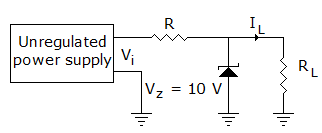# Electronics and Communication Engineering - Analog Electronics

1.

In a transistor amplifier, the reverse saturation current ICO

 A. Doubles for every 10°C rise in temperature B. Doubles for every 20°C rise in temperature C. Increase 100°C with temperature D. Doubles for every 5°C rise in temperature

Explanation:

No answer description available for this question. Let us discuss.

2.

When the emitter current of a transistor is changed by 1 mA, its collector current changes by 0.990 mA. The common-emitter short-circuit current gain is

 A. 1 B. 199 C. 99 D. 9.9

Explanation:

No answer description available for this question. Let us discuss.

3.

In figure Vi may vary from 15 to 20 V and load current may vary from 20 mA to 100 mA. Suitable maximum value of R isA. 20 Ω B. 50 Ω C. 100 Ω D. 200 Ω

Explanation:

No answer description available for this question. Let us discuss.

4.

Assertion (A): A common collector amplifier is used for matching a high impedance source to a low impedance load

Reason (R): A common collector amplifier has a near unity voltage gain

 A. Both A and R are correct and R is correct explanation for A B. Both A and R are correct but R is not correct explanation for A C. A is correct R is wrong D. A is wrong R is correct

Explanation:

No answer description available for this question. Let us discuss.

5.

In a crystal oscillator of the Colpitt's oscillator type, the crystal is put is place of the

 A. resistor R1 B. condenser C2 C. condenser C1 D. inductor L